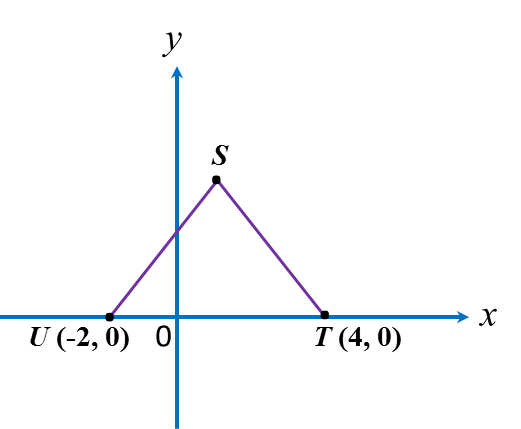# 5.2.3 Coordinates, PT3 Focus Practice

    
         
          
Question 7:
           
Diagram shows a straight line PQ on a Cartesian plane.Calculate the length, in unit, of PQ.
 
 
                
Solution:PS = 15 – 3 = 12 units
           
                
SQ = 8 – 3 = 5 units
           

By Pythagoras’ theorem,
           
PQ= PS2 + SQ2
           
= 122+ 52
           
PQ = √169
           
= 13 units
    
    
  
  
    
         
          
Question 8:
           
The diagram shows an isosceles triangle STU.Given that ST = 5 units, the coordinates of point are
            
 
   
Solution:For an isosceles triangle STU, M is the midpoint of straight line TU.
           
                       
           
Point M = (1, 0)
           

           
MT = 4 – 1 = 3 units
           
By Pythagoras’ theorem,
           
SM= ST2MT2
           
= 52 – 32
           
= 25 – 9
           
= 16
           
SM = √16
           
 = 4
           
Therefore, point S = (1, 4).